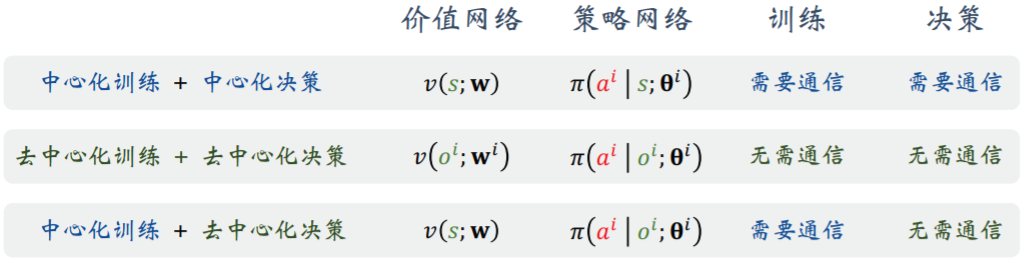0%

## 3.4 价值函数

eg. $Q_\star(s, up) = 370, Q_\star(s, left) = -120, Q_\star(s, right) = 30$, 选 up.

DQN 学习出 $Q_\star$

# 4 DQN 与 Q学习

$Q_\star(s_t, a_t) = \mathbb{E}_{s_{t+1} \sim p(\cdot | s_t, a_t)}\left[R_t + \gamma\max\limits_{a\in \mathcal{A}}Q_\star(s_{t+1}, a) \mid s_t, a_t\right]$

DQN 本质为对 $Q_\star$ 的函数近似

DQN 属于异策略 (off-policy): 用于收集经验的行为策略($\epsilon$ greedy)与控制 Agent 的目标策略不同

SARSA 属于同策略 (on-policy)

# 5 Sarsa

$Q_\pi(s_t, a_t) = \mathbb{E}_{s_{t+1},a_{t+1}}\left[R_t + \gamma Q_\pi(s_{t+1}, a_{t+1}) \mid s_t, a_t\right]$

# 6 价值学习高级技巧

### 经验回放

• 优先经验回放: 数组样本的TD误差越大, 抽取到该样本用于训练网络的概率越大 (少见 危险情况)

• 见第4部分的第一条式子, 右边的max为现有估计, 若它被高估了, 则需要更新的等式左边也会被高估
• 目标网络: 相当于新建一个 DQN, 用来算 TD 目标, 更新原 DQN, 再一定程度更新目标网络. 参数仍然与原 DQN 相关, 只能缓解自举, 不能根治.
• max本身就会使有噪声的无偏随机变量被高估
• 双Q: 用 DQN 算 $a_\star$ , 用目标网络算 TD 目标.

# 7 策略梯度

$\max\limits_{\theta} J(\theta) = \mathbb{E}_s[V_\pi(s)]$

$\nabla_\theta J(\theta)$ 的一个无偏估计为 $g(s,a;\theta) = Q_\pi(s,a) \cdot \nabla_\theta \ln \pi(a\mid s;\theta)$

$\theta \leftarrow \theta + \beta\cdot g(s,a;\theta)$​

# 8 带基线的策略梯度

### Reinforce

$g_b(s,a;\theta) = [ Q_\pi(s,a) - b ] \cdot \nabla_\theta \ln \pi(a\mid s;\theta)$

Reinforce $u_t$​ 近似 $Q_\pi(s,a)$​, 同时基于 $u_t$​​ 更新价值网络的参数

• 基于 Bellman 方程 $V_\pi(s_t) = \mathbb{E}_{A_t,S_t} [R_t + \gamma \cdot V_\pi(S_{t+1})]$

• TD 目标为 $r_t + \gamma \cdot V(s_{t+1};w)$​

• $g(s_t,a_t;\theta) = [ r_t + \gamma \cdot V(s_{t+1};w) - V(s_{t};w) ] \cdot \nabla_\theta \ln \pi(a\mid s;\theta)$​

# 9 策略学习高级技巧

### TRPO

Trust Region Policy Optimization 置信域策略优化

$J(\theta) = \mathbb{E}_S [V_\pi(S)]$

$= \mathbb{E}_S [\sum\limits_{a\in \mathcal{A}} \pi(a\mid S;\theta)Q_\pi(S,a)]$​

$= \mathbb{E}_S [\sum\limits_{a\in \mathcal{A}} \pi(a\mid S;\theta_{now})\frac{\pi(a\mid s;\theta)}{\pi(a\mid S;\theta_{now})}Q_\pi(S,a)]$​​

$= \mathbb{E}_S [\mathbb{E}_{A \sim \pi(\cdot \mid S; \theta_{now})} [\frac{\pi(A\mid S;\theta)}{\pi(A\mid s;\theta_{now})}Q_\pi(S,A)]]$​

1. 做近似
由轨迹蒙特卡洛
$L(\theta \mid \theta_{now}) = \frac{1}{n} \sum\limits_{t=1}^n \frac{\pi(a_t\mid s_t;\theta)}{\pi(a_t\mid s_t;\theta_{now})} \cdot u_t$

2. 最大化

$\max_\theta L(\theta \mid \theta_{now}); \textsf{st} ~\theta \in \mathcal{N}(\theta_{now})$

用球或 KL 散度($\pi$​ 的接近程度) 决定邻域

### 熵正则 Entropy Regularization

$\max_{\theta} ~j(\theta) + \lambda\mathbb{E}_S[H(S;\theta)]$

# 10 连续控制

### 确定策略梯度 DPG

Actor-Critic 其中策略网络的输出由各动作的概率向量变为一个确定的动作 $a = \mu(s;\theta)$

$\max_{\theta} J(\theta) = \mathbb{E}[q(s, \mu(s;\theta); w)]$

$g_j = \nabla_{\theta} q(s, \mu(s;\theta);w) = \nabla_{\theta} \mu(s;\theta)\cdot\nabla_{\mu(s;\theta)}(q(s, \mu(s;\theta);w))$

TD 算法训练价值网络

##### 双延时确定策略梯度 TD3

Twin Delayed Deep Deterministic Policy Gradient

• 1 策略网络
• 1 目标策略网络
• 2 价值网络
• 2 目标价值网络

### 随机高斯策略

$\pi(a \mid s ) = \frac{1}{\sqrt{2\pi}\sigma(s)} \cdot \exp(-\frac{[a - \mu(s)]^2}{2\sigma^2(s)})$​​​ (动作为一维)

$f(s,a;\theta) = \ln\pi(a\mid s; \theta) + \textsf{constant}$

# 十一 对状态的不完全观测

$\pi(a \mid s ; \theta) \rightarrow \pi(a \mid o_{1:t} ; \theta)$

t 可变, 即网络的输入形状可变, 不能直接使用卷积层/全连接层.

$(x_1,…,x_i) \rightarrow h_i, h_i(i=1,…,n)$ 的维度都相同, 且 $h_i$ 包含 $x_1,…,x_i$ 的所有信息, 也即最后只需保留 $h_n$​, 作为传统输入送给神经网络.​

$h_t = \tanh (W[h_{t-1};x_t]+b)$, 矩阵 $W$, 向量 $b$​ 为 RNN 的参数.​

# 十二 并行计算

2. 映射 (Map): 节点用本地数据计算 (打工仔干活)
3. 规约 (Reduce): 节点将信息发送回服务器 (打工仔交活儿)
4. 更新参数: 老板准备明天干什么活儿?
• 同步算法: 所有节点都完成映射计算后, 系统才能执行规约通信 (短板效应)
• 异步算法: 节点之间不等待, 需要服务器能够与每个节点单独通信, 接收到任一节点的结果后就更新参数, 即不同节点的参数通常是不同的.

# 十三 多智能体系统

• 合作关系 Fully Cooperative

$R_t^1 = R_t^2 = … = R_t^m, m\textsf{个Agent},~\forall t$​​

• 竞争关系 Fully Competitive

双方奖励负相关, 例如零和博弈, 奖励之和为 0

• 合作竞争混合 Mixed Cooperative & Competitive

分为多个群组, 组内合作, 组件竞争, 例如踢足球

• 利己主义 Self-Interested

只想最大化自身累计奖励

# 十四 合作关系 MARL

$S = [O^1,O^2,…,O^m]$

$R,U,Q,V,J$ 均相同

### MAC-A2C

• 价值网络: $v(s;w)$​​. 所有 Agent 共用, 输入为上方定义的 s, 输出为对 s 的评分 .TD 算法训练.

• 策略网络: $\pi(\cdot \mid s;\theta^i)$​. 每个 Agent 独有, 输入为 s, 输出为 $\mathcal{A}^i$ 的概率分布

$\nabla_{\theta^i} J(\theta^1,…,\theta^m) = \mathbb{E}_{S,A}[(Q_\pi(S,A) - V_\pi(S))\cdot\nabla_{\theta^i} \ln\pi(A^i\mid S;\theta^i)]$

$g^i(s,a;\theta^i) = (Q_\pi(s,a) - V_\pi(s))\cdot\nabla_{\theta^i} \ln\pi(a^i\mid s;\theta^i)$

$g^i(s_t,a_t;\theta^i) = (r_t+\gamma \cdot v(s_{t+1};w) - v_\pi(s_t;w))\cdot\nabla_{\theta^i} \ln\pi(a_t^i\mid s_t;\theta^i)$

Agent 不能独立做决策, 需要知道所有 Agent 的观测, 即全局状态.• 中心化训练+中心化决策: 1 个价值网络 + m 个策略网络, 中央收集各 Agent 的观测, 分配决策, Agent 完全不用思考. 由于必须等待所有观测组成状态, 产生短板效应, 延迟高.
• 去中心化训练+去中心化决策: m 个价值网络(输入为 $o^i$ 而非 $s$) + m 个策略网络(输入为 $o^i$ 而非 $s$), Agent 之间不通信, 参数独立, 本质为 SARL.
• 中心化训练+去中心化决策: 1 个价值网络 + m 个策略网络(输入为 $o^i$ 而非 $s$), 中央收集各 Agent 的观测, 训练价值网络的同时将 TD 误差广播到所有 Agent ,每个 Agent 更新自己的策略网络.

# 十五 非合作关系 MARL

$S = [O^1,O^2,…,O^m]$

$R,U,Q,V,J$​​ 不相同

$J^i(\theta^1,…,\theta^m) = \mathbb{E}[V_\pi^i(s)]$​

### MAN-A2C

Multi-Agent Non-cooperative A2C

m 个策略网络 + m 个价值网络

$\nabla_{\theta^i} J(\theta^1,…,\theta^m) = \mathbb{E}_{S,A}[(Q_\pi^i(S,A) - V_\pi^i(S))\cdot\nabla_{\theta^i} \ln\pi(A^i\mid S;\theta^i)]$​

$g^i(s_t,a_t^i;\theta^i) = (r_t^i+\gamma \cdot v(s_{t+1};w^i) - v_\pi(s_t;w^i))\cdot\nabla_{\theta^i} \ln\pi(a_t^i\mid s_t;\theta^i)$​

• 中心化训练+中心化决策: 中央收集各 Agent 的观测, m 个评委分别评价各个Agent, m 个策略网络产生动作.
• 去中心化训练+去中心化决策: 价值网络与策略网络输入为 $o^i$ 而非 $s$, Agent 之间不通信, 参数独立, 奖赏各不相同. 本质为 SARL.
• 中心化训练+去中心化决策: 策略网络输入为 $o^i$ 而非 $s$​​​​, 与 MAC-A2C 的中心化训练+去中心化决策基本相同, 区别在于有 m 个价值网络而非 1 个.

Multi-Agent Deep Deterministic Policy Gradient 多智能体深度确定策略梯度

# 十六 注意力机制与 MARL

$q^i = W_q x^i , ~k^i = W_k x^i , ~v^i = W_v x^i$

$\alpha^i = softmax[(q^i)^\top k^1,…,(q^i)^\top k^m]$

$c^i = [v^1,…,v^m]\cdot \alpha^i = \alpha_1^i v^1 + … + \alpha_m^i v^m$

$c^i$ 主要取决于最密切相关的一个或几个 $x~\rightarrow~$一个 Agent 学会判断哪些 Agent 最相关并重点关注. $c^i$ 作为全连接网络的输入.

# 十七 模仿学习

Imitation Learning

### 行为克隆

• 连续控制: 回归
• 离散控制: 多类别分类器, 输出各动作概率

### GAIL

• 生成器: $a = G(s;\theta)$, 由随机向量 $s$ 生成假样本
• 判别器: $\hat{p} = D(x;\Phi)$, 判断样本 $x$ 是真的概率
GAIL 的生成器是策略网络, 输入状态输出动作. 判别器输入状态, 输出 $|\mathcal{A}|$ 维向量 $\hat{p}$, 每个元素 $\hat{p}_a \in (0,1)$ 表示 $(s,a)$ 真假程度 ($a$ 是否为人类专家(真样本)所做)